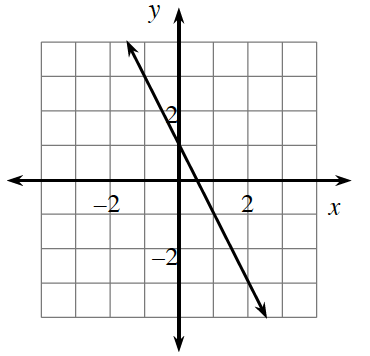### Home > INT1 > Chapter 2 > Lesson 2.2.2 > Problem2-67

2-67.Review what you know about graphs by answering the following questions.

1. What is the equation of the line graphed at right?

Use the $y$-intercept and the slope to write an equation of the line in the form of $y = mx + b$. (Create a slope triangle if you need one).

2. What are its $x$- and $y$-intercepts?

• Remember that the $x$-intercept is the point on the graph where $y = 0$ and that the $y$-intercept is the point where $x = 0$.

Use the eTool below to find the equation and determine the $x$ and $y$-intercepts.
Click the link at the right to view full version of the eTool: Int1 2-67 HW eTool.